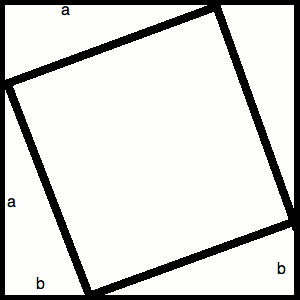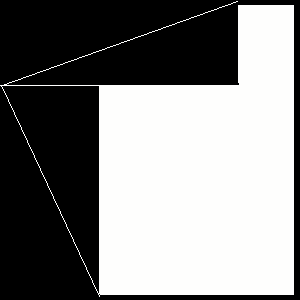# Pythagorean Theorem

The Pythagorean Theorem is the common geometric fact that the sum of the squares of the lengths of the two legs of a right triangle equals the square of the length of hypotenuse. This theorem is central to the computation of distances on a plane or in three-dimensional space, which are explored in the next module. In this module you will explore several proofs of this theorem, explaining to yourself why it is true.

## Proof Using Similar Triangles

Step 1: Draw a right triangle, and label the vertices A, B, and C, where C is the vertex of the right angle. Let a denote the length of AC, b denote the length of BC, and c denote the length of AB.

Step 2: Draw a line through vertex C that is perpendicular to the segment AB. Label the point of intersection D. Let d denote the length of CD.

Questions:
How many similar triangles are in your drawing? How do you know they are similar?
What is the ratio a/c equal to?
What is the ratio b/c equal to?
Can you get an expression for a2? For b2?

Conclusion: You should now be able to obtain the equation: a2 + b2 = c2
So you've proved the theorem!

## A More Geometric Proof

The last proof of the theorem left some things to be desired. Although it is a perfectly good proof, the final steps are shrouded in lots of algebra. One would hope that for a proof of a geometric identity, the crucial steps would rely more strongly on geometry. This proof will give you a more geometric way of thinking about the theorem. You will need two sheets of different colored construction paper, scissors, paste, and a ruler to complete the following exercise.

Step 1: Choose lengths for the variables a and b. Only use these lengths to measure things for drawing and cutting throughout the exercise. Answer all questions in terms of the variables.

Step 2: Cut a square of side lengths a+b out of one color of paper.

Step 3: Mark the point length a up the left side of the square, the point length a along the top of the square, the point length b along the bottom of the square, and the point length b up the right side of the square.

Step 4: Connect your marked points as in the following diagram, so that you have drawn four right triangles with leg lengths a and b. Label the hypotenuse of each triangle c, and fill in the missing labels for the remaining sides. Note that not all the leg lengths are labeled in the diagram.Questions:
What are the interior angles of the quadrilateral enclosed by the triangles you have drawn?
Would this be true for any values of a and b that you picked? Why or why not?
What is the area of the enclosed quadrilateral?
What is the area not covered by triangles in the original square?

Step 5:Trace your square of side lengths a+b onto the other colored sheet of paper.

Step 6: Cut the four triangles from your original square. Paste the leftmost two into their original positions on the second square, as in the image below.Step 7: Paste the remaining two triangles as in the diagram below. Label all the side lengths that you know.Questions:
What is the area of the space not covered by triangles in terms of just a and b? (Hint: It may help to subdivide the space, and find the area of each portion separately.)
Based on your earlier results in this exercise, what other value is this empty space equal to?

Conclusion: You should now be able to obtain the equation: a2 + b2 = c2
So you've proved the theorem! Again!

## Reference

"Pythagorean Theorem" (2008, August 26), (Wikipedia), Available: http://en.wikipedia.org/wiki/Pythagorean_theorem (Accessed: 2008, August 18).